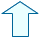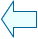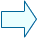PROPERTIES OF LINEAR FUNCTIONS (I) Analysis

1. DRAWING GRAPHS OF LINEAR FUNCTIONS
There are infinite points that satisfy a linear function and all these points join together to make a straight line.

y = m x

 Choose any value for m by writing in the number and then pressing Enter key or use the arrows.

1.- Check that all the points that you choose on the straight line satisfy the relation:

 y-axis coordinate = m * x-axis coordinate

2.- Change the value of m and check that all the points on the straight line satisfy the same relation.

 The graph of a linear function is always a straight line when drawn on a Cartesian coordinates system.

2. A COMMON POINT
There is one common point in all straight line graphs of linear functions.

y = m x

 Move the red point until the straight line graphs cover the whole plane.

3.- Look carefully at how all the straight line graphs of linear functions go through the origin.

4.- Also, note how there is a straight line which is not a linear function graph.

 All linear functions satisfy the following: if x = 0 then y = m*0 = 0, and therefore the coordinates (0,0) form a point on the graph. Except for the origin, all the points on the Y-axis, i.e. those points with coordinates (0,a) where a is different to 0, do not form part of a linear function graph. This is because if x is equal to 0 then y is automatically equal to 0 too.

3. DIFFERENCES BETWEEN DIFFERENT LINEAR FUNCTIONS
Each linear function has a different straight line graph.

y = m x

 Change the value of m.

5.- Note that for each value of m there is a different linear function and a different straight line graph.

6.- Which term do you think best describes the effect of m?
- the increase of the straight line
- the decrease of the straight line
- the gradient of the straight line
- the slope of the straight line
- how horizontal the straight line is
- how vertical the straight line is
- something else

 Every linear function and its graph is characterised by its value of m.

4. THE GRADIENT OF THE STRAIGHT LINE
m is usually referred to as the gradient of the line.

y = m x

 Change the value of m.

7.- Analyse what happens to the graph when the gradient has a large value, a value close to 0 and a negative value. Write a conclusion in your notebook.

 The value of the gradient determines the slope of the line. A value close to 0 makes the line more horizontal whereas greater values make the line more vertical.Juan Madrigal MugaSpanish Ministry of Education. Year 2001